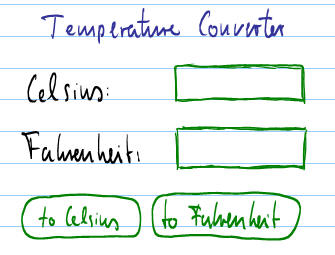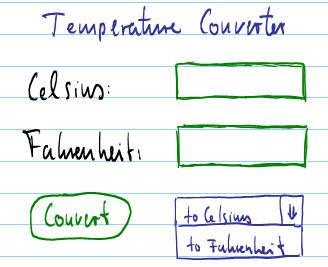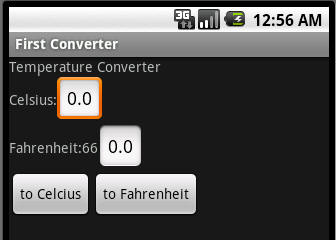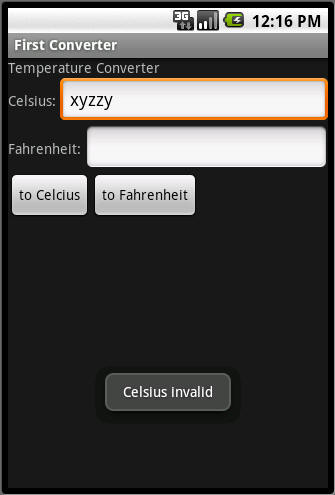#MathCS.org - Android

## A Temperature Converter App

java -> android -> temp converter ...

In this segment we will create a fully functioning program that uses labels, input fields, and buttons to convert temperatures between degrees Celsius and Fahrenheit. First, let's get the math out of the way:

• to convert C degrees Celsius into degrees Fahrenheit:
• F = 9/5 * C + 32
• to convert F degrees Fahrenheit into degrees Celsius:
• C = 5/9 * (F - 32)

When creating the program, the eternal question is what to worry about first: form or function. In other words, should you first design the layout, then worry about how the program works, or should you create the inner workings first, then design a perfect look. It's little like the chicken and egg problem but usually an iterative process works well:

• create a rough layout with all important components arranged in a functional way
• implement how the program logic works
• refine the layout, then refine the logic, then the layout, then the logic, etc.

So, let's sketch out the look of the program. We might try something like this:orLet's try to create the look on the left: we need a label as title in the top row, second and third row contain a label followed by an input field each, and last there are two buttons in sequence. We'll use one vertical linear layout containing four horizontal linear layouts that in turn contain the widgets (defined as fields). First, as usual we create a new project in Eclipse:

• Select "File | New | Project" and pick "Android Project"
• Click "Next" and give it a name, say "FirstConverter"
• Check the "Google 1.6" platform
• Use "Temperature Converter" as Application name
• Use your standard package prefix, e.g. org.mathcs.firstinteract (or leave blank)
• Check "Create Activity" and name it "FirstConverter"
• Click "Finish"

The code to create this layout, approximately, goes like this:

```public class FirstConverter extends Activity
{
private Button toCelsiusButton = null;
private Button toFahrenheitButton = null;
private EditText celsiusField = null;
private EditText fahrenheitField = null;
private TextView titleLabel = null;
private TextView celsiusLabel = null;
private TextView fahrenheitLabel = null;

public void onCreate(Bundle savedInstanceState)
{
super.onCreate(savedInstanceState);

// initializing the widgets
toCelsiusButton = new Button(this);
toFahrenheitButton = new Button(this);
celsiusField = new EditText(this);
fahrenheitField = new EditText(this);
titleLabel = new TextView(this);
celsiusLabel = new TextView(this);
fahrenheitLabel = new TextView(this);

// setting the text the widgets should display
toCelsiusButton.setText("to Celcius");
toFahrenheitButton.setText("to Fahrenheit");
celsiusField.setText("0.0");
fahrenheitField.setText("0.0");
titleLabel.setText("Temperature Converter");
celsiusLabel.setText("Celsius: ");
fahrenheitLabel.setText("Fahrenheit: ");

// laying out the widgets using the easiest layout manager
LinearLayout row1 = new LinearLayout(this);
LinearLayout row2 = new LinearLayout(this);
LinearLayout row3 = new LinearLayout(this);
LinearLayout row4 = new LinearLayout(this);
LinearLayout mainLayout = new LinearLayout(this);
mainLayout.setOrientation(LinearLayout.VERTICAL);

// setting the view to the "master" layout
setContentView(mainLayout);
}
}```

This will generate the following look, which is not perfect but good enough for our first iteration:We can now worry about making the program work, since currently the buttons don't do anything when tapped. Our first iteration might go as follows:

• If the "to Celsius" button is clicked:
• retrieve the value from the "Fahrenheit" field
• convert it to Celsius
• put the answer into the "Celsius" field
• If the "to Fahrenheit" button is clicked:
• retrieve the value from the "Celsius" field
• convert it to Fahrenheit
• put the answer into the "Fahrenheit" field

We know how to activate buttons (by adding inner classes as handlers and registering them to the respective buttons), so we create the following inner classes as fields:

```	private class ToCelsiusHandler implements View.OnClickListener
{
public void onClick(View v)
{
convertToCelsius();
}
}

private class ToFahrenheitHandler implements View.OnClickListener
{
public void onClick(View v)
{
convertToFahrenheit();
}
}```

The actual work of these handlers is done in the convertToCelsius and convertToFahrenheit methods. Of course we need to add those methods (to the end of our class):

```	private void convertToCelsius()
{
String fahrenheitString = fahrenheitField.getText().toString();
double f = Double.parseDouble(fahrenheitString);
double c = 5.0/9.0 * (f - 32.0);
celsiusField.setText(String.valueOf(c));
}

private void convertToFahrenheit()
{
String celsiusString = celsiusField.getText().toString();
double c = Double.parseDouble(celsiusString);
double f = 9.0/5.0 * c + 32.0;
fahrenheitField.setText(String.valueOf(f));
}```

We use the static conversion methods Double.parseDouble and String.valueOf to convert data types from String to double and conversely. To register these handlers, we register them as listeners to the respective buttons by adding the following lines to the end of the onCreate method:

```        toCelsiusButton.setOnClickListener(new ToCelsiusHandler());
toFahrenheitButton.setOnClickListener(new ToFahrenheitHandler());```

When we now run this program, it should work - in principle. But:

• When you enter numbers in the fields, they expand to accommodate additional digits. It would look nicer if the fields span a row right from the start so they would not need to expand. Thus, we need to improve the look.
• When you, say by accident, enter a string or characters into a field and try to convert, your program crashes (force quit). Hence, we need to improve the logic.

We are on for our second iteration. To improve the look we need to use "attributes" when adding the widgets to the layout. This is much easier using XML, which we will do in the next segment, but for now we'll do it programmatically: There are several addView methods for a layout (the method is overloaded). We already saw the one which accepts a View such as an EditText as input, but a second variant accepts a view and a set of layout parameters. We will use it to add the editable fields celsiusField and fahrenheitField with a default width of FILL_PARENT (to take all remaining space) and height WRAP_CONTENT (to use as much height as necessary). In other words, we replace the lines row2.addView(celsiusField) with:

`        row2.addView(celsiusField, new ViewGroup.LayoutParams(LayoutParams.FILL_PARENT, LayoutParams.WRAP_CONTENT));`

`        row3.addView(fahrenheitField, new ViewGroup.LayoutParams(LayoutParams.FILL_PARENT, LayoutParams.WRAP_CONTENT)); `

That changes the layout to the more acceptable look:There is still room for improvement (title could be larger font and bold, the two buttons could be centered, etc.) but we'll instead focus on the logic now. After all, a program that crashes is worse than one that does not look optimal.

The crash occurs when a user enters a string or character into the field that does not represent a valid double number. When we then use the Double.parseDouble method, it throws an exception that does not get caught, which crashes our program! We therefore add error handling code to both conversion methods, where we use a Toast to inform the user that a number entered does not represent a valid number. A Toast is a short message that appears briefly on the screen and disappears after a short time. It is a very convenient way to inform the user without being intrusive. It can be created most conveniently by using the static makeToast method.Here are the new conversion methods (that replace the old ones), using a 'toast' to let the user know if something went wrong:

```	private void convertToCelsius()
{
try
{
String fahrenheitString = fahrenheitField.getText().toString();
double f = Double.parseDouble(fahrenheitString);
double c = 5.0/9.0 * (f - 32.0);
celsiusField.setText(String.valueOf(c));
}
catch(Exception ex)
{
Toast.makeText(this, "Fahrenheit invalid", Toast.LENGTH_SHORT).show();
}
}

private void convertToFahrenheit()
{
try
{
String celsiusString = celsiusField.getText().toString();
double c = Double.parseDouble(celsiusString);
double f = 9.0/5.0 * c + 32.0;
fahrenheitField.setText(String.valueOf(f));
}
catch(Exception ex)
{
Toast.makeText(this, "Celsius invalid", Toast.LENGTH_SHORT).show();
}
}```

If you now try to convert an invalid number, the program now informs the user briefly that something is wrong without crashing:At this point we could go back and improve the look further. However, that would involve adding quite a number of attributes to the layouts and views, which is possible but tedious. We will instead learn a much more convenient way to specify how a program should look in our next segment.